# MCAT Physical : Oxidation-Reduction

## Example Questions

← Previous 1 3

### Example Question #1 : Oxidation Reduction

Consider the redox reaction: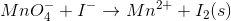What is the balanced equation for the above redox reaction in acidic solution?

Possible Answers: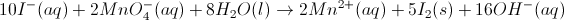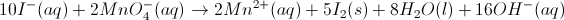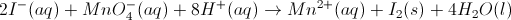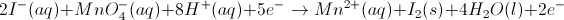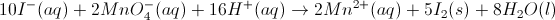Correct answer:Explanation:1. Determine the oxidation number of each element.

Reactants: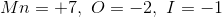Products: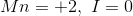2. Write and balance the half reactions.

Oxidation (loss of electrons):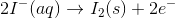Reduction (gain of electrons):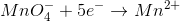3. Balance oxygen by adding water (from the solution) into the half reactions.

Oxidation:Reduction: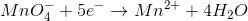4. Balance hydrogen by adding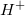ions from the acid into the half reactions.

Oxidation:Reduction: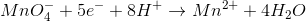5. Multiply each half reaction times an integer such that the electrons cancel when the equations are added. The oxidation reaction will be multiplied by five, and the reduction reaction will be multiplied by two.

Oxidation times 5: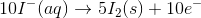Reduction times 2: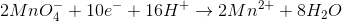6. Add the half reactions together. The electrons will cancel from both the reactants and products.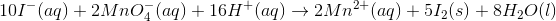### Example Question #2 : Oxidation Reduction

Consider the half reaction below.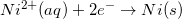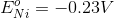Which of the following statements is true about the oxidation of nickel?

Possible Answers:

The oxidation of nickel has a potential of 0V

The oxidation of nickel has a potential of 0.23V

The nickel will gain electrons when oxidized

It is not possible to oxidize nickel

None of these are true

Correct answer:

The oxidation of nickel has a potential of 0.23V

Explanation:

Reduction potentials are typically provided in table form. Since the oxidation potential of an element is the negative of the reduction potential, it can be determined by viewing a reduction table.

Since the reduction potential for nickel is given as –0.23V, we can conclude that the oxidation potential for nickel is 0.23V.

### Example Question #2 : Reduction Potentials

The standard reduction potentials for iron and nickel are as follows: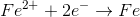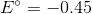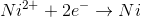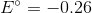If a galvanic cell is made using these two metals, what is the standard voltage for the cell?

Possible Answers: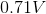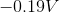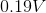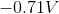Correct answer:Explanation:

A galvanic cell is spontaneous, meaning that there will be a positive cell voltage. In order for this to take place, the more negative reduction potential must be flipped so that the metal is oxidized.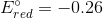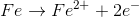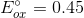Adding these values together gives a standard cell voltage of.

### Example Question #1 : Oxidation Number

What is the oxidation state of sulfur in H2SO4?

Possible Answers:

+6

-2

+4

-5

0

Correct answer:

+6

Explanation:

Since there is no overall charge on the compound the oxidation states must cancel out. Although the oxidation state of hydrides are +1, there are two in the compound that must be accounted for. The same is true for oxygen; although the oxidation number of oxygen is -2, there are four oxygens present accounting for a total of -8. The +2 state contribution from the hydrides and -8 from the oxygens results in a -6 charge. The oxidation state on sulfur must be +6 for the molecule to be neutral.

### Example Question #1 : Oxidation Number

What is the oxidation number of chromium in calcium dichromate (CaCr2O7)?

Possible Answers:

+2

-2

+6

0

+7

Correct answer:

+6

Explanation:

Since the calcium ion has a charge of +2, the dichromate anion must have a charge of -2. Oxygen always has an oxidation number of -2, so the seven oxygens in the molecule have a total number of -14. That leaves a charge of +12 that the two chromiums must balance out (-2 - (-14) = 12). Each chromium must therefore have an oxidation number of +6.

### Example Question #1 : Oxidation Number

What is the oxidation state of nitrogen in HNO3?

Possible Answers: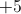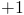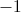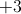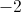Correct answer:Explanation:

When applying oxidation numbers there are certain hierarchical rules that must be followed.

1. The sum of oxidation states of all the elements in a molecule must add up to the overall charge.

2. Group 1 and Group 2 elements have +1 and +2 oxidation states, respectively.

3. Fluorine has an oxidation state of –1.

4. Hydrogen has an oxidation state of +1 (except in metal hydrides).

5. Oxygen has an oxidation state of –2.

6. Elements of the same group (excluding transition metals) generally have the same oxidation state.

When applying the oxidation state to HNO3, hydrogen has a +1 oxidation state, and each of the oxygen molecules has a –2 oxidation state. Since there is only one hydrogen molecule and three oxygen molecules, the oxidation state of nitrogen must balance out the charge of the hydrogen molecule and oxygen molecules combined.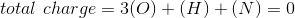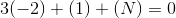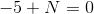Nitrogen must have a +5 oxidation state.

### Example Question #1 : Oxidation Number

What is the oxidation number of hydrogen in NaH?

Possible Answers: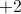Correct answer:Explanation:

When applying oxidation numbers there are certain hierarchical rules that must be followed.

1. The sum of oxidation states of all the elements in a molecule must add up to the overall charge.

2. Group 1 and Group 2 elements have +1 and +2 oxidation states, respectively.

3. Fluorine has an oxidation state of –1.

4. Hydrogen has an oxidation state of +1 (except in metal hydrides).

5. Oxygen has an oxidation state of –2.

6. Elements of the same group (excluding transition metals) generally have the same oxidation state.

Following the oxidation rules, group 1 elements must have an oxidation number of +1.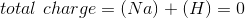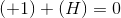To balance out the overall charge of zero, the hydrogen in this compound must have an oxidation state of –1. Note that NaH is a metal hydride; therefore, the oxidation state of hydrogen is not necessarily +1.

### Example Question #3 : Oxidation Number

What is the correct oxidation number of zinc in the compound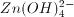?

Possible Answers:

0

-1

+2

+1

-2

Correct answer:

+2

Explanation:

The sum of oxidation numbers of each element in this compound must add to the total charge of -2. Hydrogen always has an oxidation number of +1, and oxygen always has an oxidation number of -2.

So, in total for oxygen we have (4) * (-2) = -8, and for hydrogen we have (4) * (+1) = +4.

Combining the oxygen and hydrogen, (+4) + (-8) = -4, so we need an additional +2 to achieve the total charge of -2. Zn must provide this balancing charge, and have an oxidation number of +2.

### Example Question #1 : Oxidation Number

Which of the following statements between HClO4 and HClO3 is true?

Possible Answers:

The oxidation number for chlorine in HClO4 has increased in HClO3

The oxidation number of oxygen in HClO4 has been decreased in HClO3

The oxidation numbers for all atoms are the same in both molecules

The oxidation number of chlorine in HClO4 has been decreased in HClO3

Correct answer:

The oxidation number of chlorine in HClO4 has been decreased in HClO3

Explanation:

Oxidation number is a concept that you will also encounter in electrochemistry lectures. It is used to track electrons in an oxidation-reduction reaction. Overall, the sum of the oxidation states of all of the atoms in the molecule must equal the overall charge on the molecule. It is important to remember some of the commonly accepted oxidation states for atoms, so that you can predict the oxidation numbers on other atoms. Here are some key oxidation numbers useful to know for the MCAT:

1. Atoms in elemental form have an oxidation state of 0.

2. Halogens are commonly given an oxidation state of –1.

3. Hydrogen and alkali metals are commonly given an oxidation state of +1.

4. Oxygen is commonly given an oxidation number of –2.

Keeping these rules in mind, we can assume that the hydrogens and oxygens in the two compounds maintain the same oxidation state. Chlorine, however, is attached to one less oxygen in HClO3. As a result, the oxidation state of Cl has decreased from +7 to +5, when comparing HClO4 to HClO3.

### Example Question #1 : Oxidation Number

Which element in the given compounds has the highest oxidation number?

Possible Answers:

Carbon in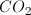Aluminum in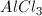Sulfur in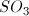Sulfur in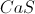Oxygen in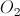Correct answer:

Sulfur inExplanation:

We can calculate the oxidation number in each answer to find the greatest value.

In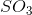, oxygen will have a value ofand the molecule is neutral. Sulfur must have an oxidation number of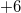to balance the three oxygens.

In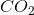, oxygen will have a value ofand the molecule is neutral. Carbon must have an oxidation number of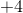to balance the two oxygens.

In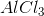, chlorine will have a value ofand the molecule is neutral. Aluminum must have an oxidation number of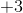to balance the three chlorines.

In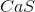, calcium will have a value ofand the molecule is neutral. Sulfur must have an oxidation number of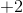to balance the calcium.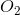is a molecule comprised of a single element; each atom will have an oxidation number of.

← Previous 1 3

### All MCAT Physical Resources